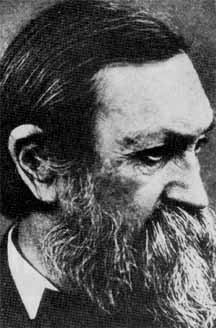Mathematical and Numerical aspects of
Low Mach Number Flows
June 21-25, 2004 / Porquerolles, France
It's over !

Some Slides of the presentation are available Here#### Low Mach number flows represent a significant part of the various flows encountered in geophysics, industry or every day life. Paradoxically, the mathematical analysis of the equations governing these flows is difficult and on the practical side, the research of numerical algorithms valid for all flow speeds is continuing to be a challenge. However, in the last decade, both from the theoretical and the numerical sides, significant progresses were made in the understanding and analysis of the equations governing these flows. This conference intends to provide an up-to-date inventory of recent mathematical and numerical results in the analysis of these flows by bringing together both mathematicians and numericists active in this area.

In addition to plenary lectures and tutorials given by well known experts of the field, the program will include contributed talks of thirty minutes. Papers on the mathematical and numerical analysis and modelling of Low Mach number flows are sought. One page abstracts should be submitted by February 29 2004. The program will also include a numerical workshop proposing to compute several challenging low Mach number flows